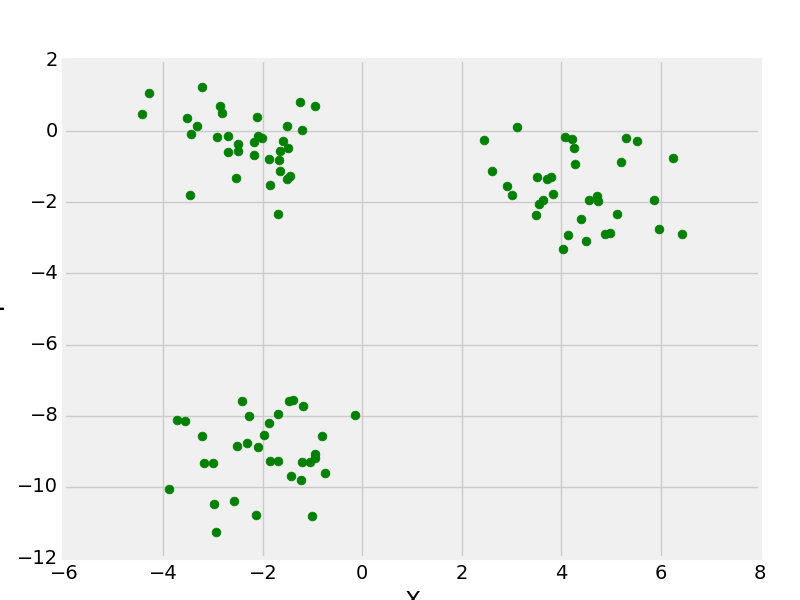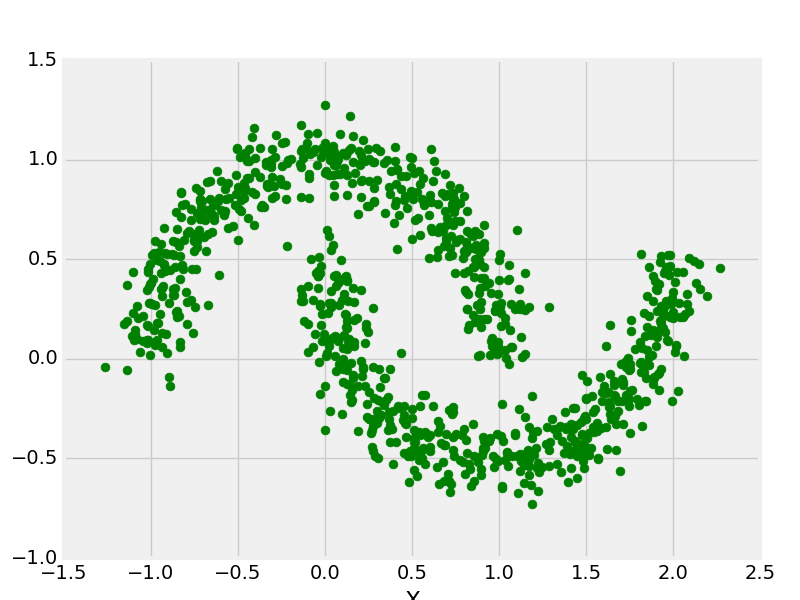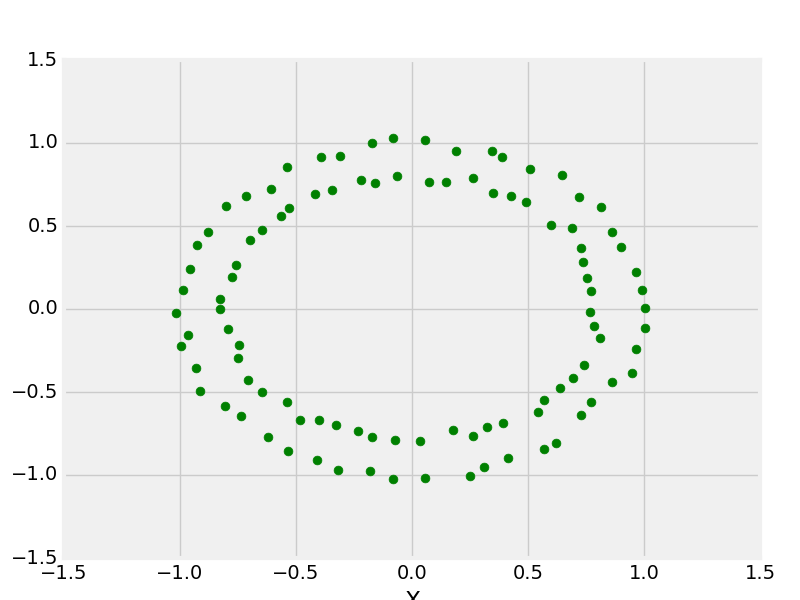# Python | Create Test DataSets using Sklearn

Python’s Sklearn library provides a great sample dataset generator which will help you to create your own custom dataset. It’s fast and very easy to use. Following are the types of samples it provides.
For all the above methods you need to import sklearn.datasets.samples_generator

## Python3

 `# importing libraries` `from` `sklearn.datasets ``import` `make_blobs`   `# matplotlib for plotting` `from` `matplotlib ``import` `pyplot as plt ` `from` `matplotlib ``import` `style`

## Python3

 `# Creating Test DataSets using sklearn.datasets.make_blobs` `from` `sklearn.datasets ``import` `make_blobs` `from` `matplotlib ``import` `pyplot as plt ` `from` `matplotlib ``import` `style`   `style.use(``"fivethirtyeight"``)`   `X, y ``=` `make_blobs(n_samples ``=` `100``, centers ``=` `3``, ` `               ``cluster_std ``=` `1``, n_features ``=` `2``)`   `plt.scatter(X[:, ``0``], X[:, ``1``], s ``=` `40``, color ``=` `'g'``)` `plt.xlabel(``"X"``)` `plt.ylabel(``"Y"``)`   `plt.show()` `plt.clf()`

Output:make_blobs with 3 centers

## Python3

 `# Creating Test DataSets using sklearn.datasets.make_moon` `from` `sklearn.datasets ``import` `make_moons` `from` `matplotlib ``import` `pyplot as plt ` `from` `matplotlib ``import` `style`   `X, y ``=` `make_moons(n_samples ``=` `1000``, noise ``=` `0.1``)` `plt.scatter(X[:, ``0``], X[:, ``1``], s ``=` `40``, color ``=``'g'``)` `plt.xlabel(``"X"``)` `plt.ylabel(``"Y"``)`   `plt.show()` `plt.clf()`

Output:make_moons with 1000 data points

## Python3

 `# Creating Test DataSets using sklearn.datasets.make_circles` `from` `sklearn.datasets ``import` `make_circles` `from` `matplotlib ``import` `pyplot as plt ` `from` `matplotlib ``import` `style`   `style.use(``"fivethirtyeight"``)`   `X, y ``=` `make_circles(n_samples ``=` `100``, noise ``=` `0.02``)` `plt.scatter(X[:, ``0``], X[:, ``1``], s ``=` `40``, color ``=``'g'``)` `plt.xlabel(``"X"``)` `plt.ylabel(``"Y"``)`   `plt.show()` `plt.clf()`

Output:make _circle with 100 data points

Scikit-learn (sklearn) is a popular machine learning library for Python that provides a wide range of functionalities, including data generation. In order to create test datasets using Sklearn, you can use the following code:

### Advantages of creating test datasets using Sklearn:

1. Time-saving: Sklearn provides a quick and easy way to generate test datasets for machine learning tasks, which saves time compared to manually creating datasets.
2. Consistency: The datasets generated by Sklearn are consistent and reproducible, which helps ensure consistency in your experiments and results.
3. Flexibility: Sklearn provides a wide range of functions for generating datasets, including functions for classification, regression, clustering, and more, which makes it a flexible tool for generating test datasets for different types of machine learning tasks.
4. Control over dataset parameters: Sklearn allows you to customize the generation of datasets by specifying parameters such as the number of samples, the number of features, and the level of noise, which gives you greater control over the test datasets you create.

### Disadvantages of creating test datasets using Sklearn:

1. Limited dataset complexity: The datasets generated by Sklearn are typically simple and may not reflect the complexity of real-world datasets. Therefore, it may not be suitable for testing the performance of machine learning algorithms on complex datasets.
2. Lack of diversity: Sklearn datasets may not reflect the diversity of real-world datasets, which may limit the generalizability of your machine learning models.
3. Overfitting risk: If you generate test datasets that are too similar to your training datasets, there is a risk of overfitting your machine learning models, which can result in poor performance on new and unseen data.
4. Overall, Sklearn provides a useful tool for generating test datasets quickly and efficiently, but it’s important to keep in mind the limitations and potential drawbacks of using synthetic datasets for machine learning testing. It’s recommended to use real-world datasets whenever possible to ensure the most accurate representation of the problem you are trying to solve.

Whether you're preparing for your first job interview or aiming to upskill in this ever-evolving tech landscape, GeeksforGeeks Courses are your key to success. We provide top-quality content at affordable prices, all geared towards accelerating your growth in a time-bound manner. Join the millions we've already empowered, and we're here to do the same for you. Don't miss out - check it out now!

Previous
Next